# Community

Blog Events Webinars Tutorials Forum
×
Community Blog Code Explanation of MySQL 8.0.23 Hypergraph Join Optimizer

# Code Explanation of MySQL 8.0.23 Hypergraph Join Optimizer

This article gives a deep code explanation of MySQL 8.0.23 Hypergraph Join Optimizer.

By Daoke

## MySQL Join

MySQL itself does not have a conventional execution plan. Instead, it is typically composed of two structures: JOIN and QEP_TAB (Query Execution Plan Table). QEP_TAB can represent various types of tables, such as physical tables, memory tables, constant tables, and result tables from subqueries. As the carrier of the entire independent JOIN execution plan, it is responsible for invoking and forwarding the entire execution path. However, starting from MySQL 8.0.20, an independent Iterator executor engine mode has been fully developed. With the introduction of the AccessPath concept in MySQL 8.0.22, a true independent execution plan is possible, further optimizing the process from a tree execution plan to the execution of the Iterator carrier in the execution engine.

MySQL's original Join relies on the QEP_TAB list because it does not support other forms of Join structures and only supports left-deep trees, which can be represented directly with arrays. When the optimizer generates an execution plan, it simply needs to add the JOIN attribute, op_type, to QEP_TAB. This allows for recursive use of different Join methods and table access methods.

``````// Operation between the previous QEP_TAB and this one.
enum enum_op_type {
// Regular nested loop.
OT_NONE,

// Aggregate (GROUP BY).
OT_AGGREGATE,

// Various temporary table operations, used at the end of the join.
OT_MATERIALIZE,
OT_AGGREGATE_THEN_MATERIALIZE,
OT_AGGREGATE_INTO_TMP_TABLE,
OT_WINDOWING_FUNCTION,

// Block-nested loop (rewritten to hash join).
OT_BNL,

// Batch key access.
OT_BKA
} op_type = OT_NONE;``````

## Hypergraph Join Optimizer

There are 11 official Patches to submit enhancements to the Join optimizer, including a significant reconstruction of the optimizer and executor. Let's first look at how the official submits such a major reconstruction.

### [Basic] DPhyp-Hypergraph Partitioning Algorithm

The official team initially implemented the DPhyp-Hypergraph partitioning algorithm. For more details, please refer to the paper titled "Dynamic Programming Strikes Back." There are many Join ordering algorithms in the database, and the authors of the second and third articles in the reference have explained them in detail. Here, I will provide a brief introduction.

Each query can be defined as an undirected query graph. All relations R1, R2, ..., Rn in the query are considered as nodes. The join predicate expressions are represented as edges, such as a1 = a2 (where a1 ∈ Ri and a2 ∈ Rj). Join predicates containing constants form self-edges, for example, a1 = const (where a1 ∈ Ri). Most self-edges are not taken into consideration in the Join algorithm as they are pushed down. For example, for `select * from Student s, Attend a, Lecture l, Professor p where s.sno = a.asno and a.alno = l.lno and l.lpno = p.pno and p.pname = 'Larson'`, the query graph structure is as follows: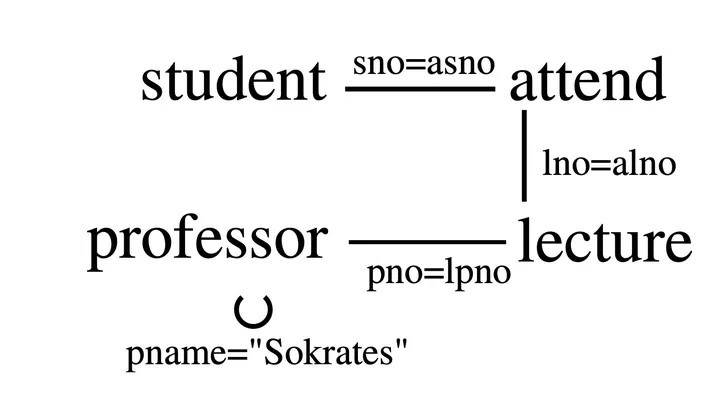Join trees have the following types: left-deep tree, right-deep tree, zigzag tree, and bushy tree. The first three are linear Join trees. MySQL used to adopt left-deep trees. To better support Hash Join and NestLoop Join, it decides to adopt bushy trees. To avoid Cartesian product Join at any time, the Join ordering algorithm for linear Join is simple. So why do we introduce the complex bushy tree? Assume that Query (R1, R2, R3) has the following attributes: y | R1 | = 10, | R2 | = 20, | R3 | = 20, | R4 | = 10, f1,2 = 0.01, f2,3 = 0.5, f3,4 = 0.01. | | represents the number of rows, and fn,m represents the selection rate of Rn and Rm. You can see that bushy tree has better execution efficiency.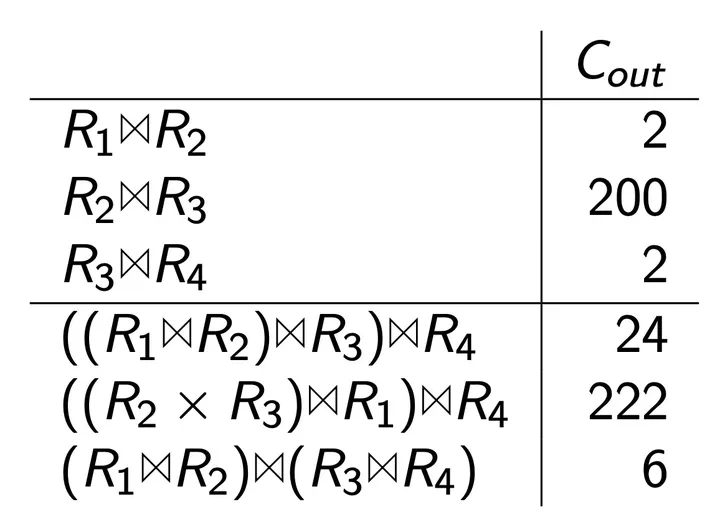Unfortunately, the bushy tree has a higher search possibility: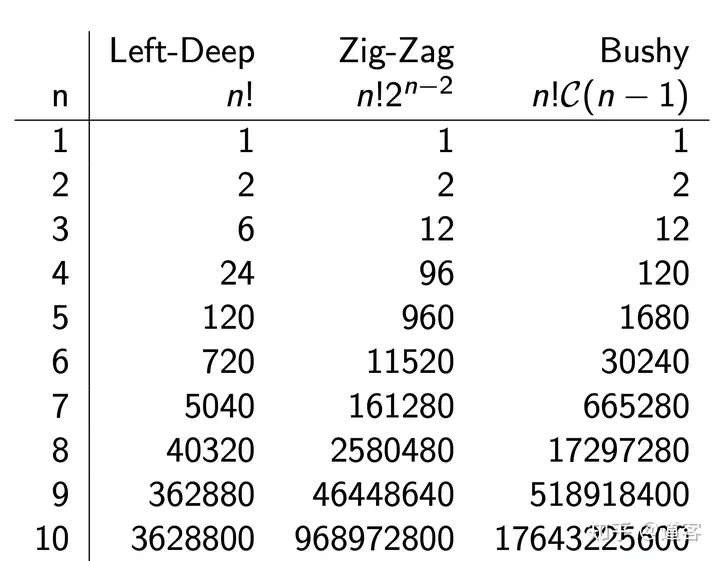Therefore, the original left-deep tree uses the Greedy Heuristics algorithm. For the bushy tree, the calculation of Join Ordering usually uses a dynamic programming algorithm (DPccp and DPhyp).

The DPccp algorithm is as follows: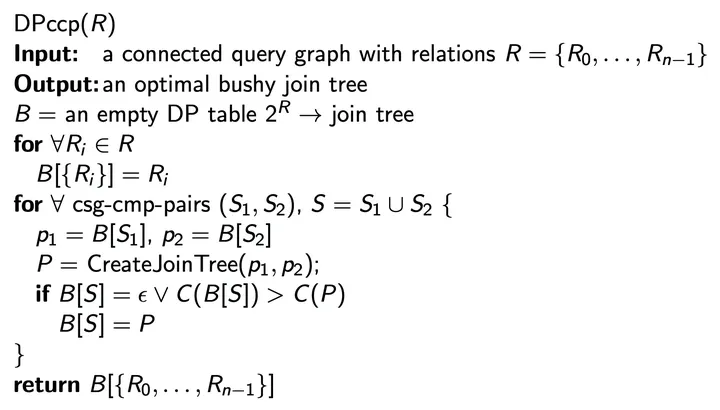However, DPccp has many limitations: Predicates are complex and involve multiple tables (R1, R2, R3) as joins, for example, R1.a + R2.b + R3.c = R4.d + R5.e + R6.f. Only inner joins are supported. Therefore, a new Hypergraph-based algorithm, DPhyp, is introduced.

``````select *
from R1 r1, R2 r2, R3 r3,
R4 r4, R5 r5, R6 r6
where r1.a=r2.a and r2.b=r3.c and
r4.d=r5.d and r5.e=r6.e and
abs(r1.f + r3.f )
= abs(r4.g + r6.g)``````

Before introducing the algorithm, I'll introduce the basic concept of hypergraph. Compared to an ordinary graph, its characteristic is that the node in the graph is a set, which is called a hypernode, and the edge of the graph joins the hypernodes, which equals joining two sets. Such edges are called hyperedges. Hypergraph is composed of the most basic elements: hypernodes and hyperedges. With a hypergraph, the above Join Graph can be changed into: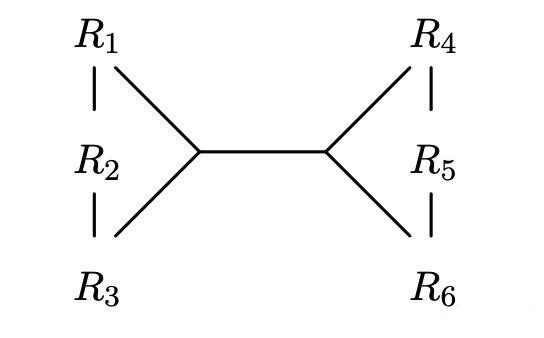Due to the use of DPccp and Top-Down Partition Search, the algorithms of outer join and antijoin that cannot be rearranged freely cannot be solved.

MySQL is represented with Bitmap (64bit). Assume that the number of Join tables will not exceed 61. The definition is as follows.

``````+struct Hyperedge {
+  // The endpoints (hypernodes) of this hyperedge. See the comment about
+  // duplicated edges in Node.
+  //
+  // left and right may not overlap, and both must have at least one bit set.
+  NodeMap left;
+  NodeMap right;
+};
+
+struct Hypergraph {
+  std::vector<Node> nodes;  // Maximum 8*sizeof(NodeMap) elements.
+  std::vector<Hyperedge> edges;
+
+  void AddEdge(NodeMap left, NodeMap right);
+};``````

The basic algorithm process is as follows:

1. Find the seed node Ri in the graph.
2. Continue to increase i to find hyperedges, regardless of unconnected and processed ones.
3. For each connected subgraph (csg), repeat steps 1 and 2 to find another connected subgraph (complement, cmp), and then connect the cmp of this graph to become a larger connected subgraph (csg-cmp-pair).
4. When a csg-cmp-pair is found, a subjoin that can be estimated is formed.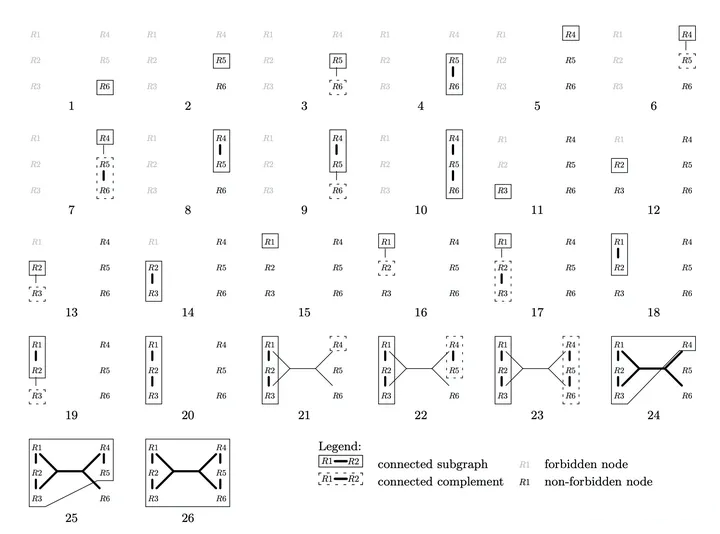If you are interested in this section, you can read related papers and MySQL code (sql/join_optimizer).

### QEP_TAB and Executor Iterator Are Decoupled to Reset the InnoDB row buffer.

As we all know, the QEB_TAB structure carries a lot of information. In addition to the information about table access and Join methods, there are also InnoDB row buffer, optimized access modes of table access (ref/range/loose scan/first match/materialize), additional attributes (having/distinct/sort/icp/later derived/mrr/cte), and basic physical table structures such as TABLE_LIST. If you need to delete QEP_TAB, the first thing is to decouple it from the executor Iterator.

``````class TableScanIterator final : public TableRowIterator {
public:
-  // Accepts nullptr for qep_tab; qep_tab is used only for setting up record
-  // buffers.
-  //
-  // The pushed condition can be nullptr.
+  // “expected_rows” is used for scaling the record buffer.
+  // If zero or less, no record buffer will be set up.
//
// "examined_rows", if not nullptr, is incremented for each successful Read().
-  TableScanIterator(THD *thd, TABLE *table, QEP_TAB *qep_tab,
+  TableScanIterator(THD *thd, TABLE *table, double expected_rows,
ha_rows *examined_rows);``````

Next, decouple.

``````-static bool init_index_and_record_buffer(const QEP_TAB *qep_tab, handler *file,
+static bool init_index(TABLE *table, handler *file, uint idx, bool sorted) {

-bool set_record_buffer(const QEP_TAB *tab);
+bool set_record_buffer(TABLE *table, double expected_rows_to_fetch);

=>

-  return init_index_and_record_buffer(m_qep_tab, m_qep_tab->table()->file,
-                                      m_ref->key, m_use_order);
+  if (table()->file->inited) return false;
+  if (init_index(table(), table()->file, m_ref->key, m_use_order)) {
+    return true;
+  }
+  return set_record_buffer(table(), m_expected_rows);``````

### Make CostingReceiver and Transform Query Block select_lex become Hypergraph

MySQL 8.0.23 provides the first prototype version of the optimizer model that supports hypergraph, which is opened with set optimizer_switch="hypergraph_optimizer=on". Its main differences from the original optimizer are:

• The execution plans are no longer limited to the left-deep tree.
• The DPhyp dynamic programming algorithm replaces the powerful calculation and heuristic pruning method, reducing the search space. However, there are some limitations.
• Hash join becomes the main choice.
• It directly interconnects with AccessPath instead of directly generating Iterators.

This is mainly implemented by FindBestQueryPlan function. The logic is as follows:

• First determine whether the query syntax (CheckSupportedQuery) is supported by the new optimizer. If it is not supported, an error, ER_HYPERGRAPH_NOT_SUPPORTED_YET, is returned directly.
• Convert top_join_list to a JoinHypergraph structure. Since Hypergraph is a relatively independent algorithm-level implementation, the JoinHypergraph structure is used to better encapsulate the structure of the database into the concepts of Hypergraph edges and nodes.
• The DPhyp algorithm in the paper is implemented by EnumerateAllConnectedPartitions.
• The CostingReceiver class contains the main logic of previous JOIN planning, including selecting the corresponding access path based on cost, evaluating the sub-plan generated by DPhyp, and retaining the sub-plan with the smallest cost.
• After the root_path is obtained, handle group/agg/having/sort/limit. For Group BY operation, Hypergraph uses sorting first + streaming aggregation.

Finally, the corresponding execution Iterator tree is generated by using the CreateIteratorFromAccessPath function, which is executed in the Iterator executor.

### Examples:

``````Two connected subgraphs
root:test> explain format=tree select * from t1,t2,t3,t4 where t2.f2 = t1.a and t1.a = t3.a;
+------------------------------------------------------------------------------------------------------------------------------------------------------------------------------------------------------------------------------------------------------------------------------------------------------------------------------------------------------------------------------------------------------------------------------------------------------------------+
| EXPLAIN                                                                                                                                                                                                                                                                                                                                                                                                                                                          |
+------------------------------------------------------------------------------------------------------------------------------------------------------------------------------------------------------------------------------------------------------------------------------------------------------------------------------------------------------------------------------------------------------------------------------------------------------------------+
| -> Hash cartesian product (no condition)  (cost=1.83 rows=2)
-> Inner hash join (t2.f2 = t1.a)  (cost=1.55 rows=2)
-> Table scan on t2  (cost=0.25 rows=2)
-> Hash
-> Inner hash join (t1.a = t3.a)  (cost=1.27 rows=1)
-> Table scan on t1  (cost=1.00 rows=1)
-> Hash
-> Table scan on t3  (cost=0.25 rows=1)
-> Hash
-> Table scan on t4  (cost=0.25 rows=1)
|

A connected subgraph
root:test> explain format=tree select * from t1,t2,t3,t4 where t2.f2 = t1.a and t1.a = t3.a and t2.f2 = t4.pk and t1.a = t4.pk;
+----------------------------------------------------------------------------------------------------------------------------------------------------------------------------------------------------------------------------------------------------------------------------------------------------------------------------------------------------------------------------------------------------------------------------------------------------------------------------+
| EXPLAIN                                                                                                                                                                                                                                                                                                                                                                                                                                                                    |
+----------------------------------------------------------------------------------------------------------------------------------------------------------------------------------------------------------------------------------------------------------------------------------------------------------------------------------------------------------------------------------------------------------------------------------------------------------------------------+
| -> Inner hash join (t2.f2 = t4.pk), (t1.a = t4.pk)  (cost=1.83 rows=2)
-> Inner hash join (t2.f2 = t1.a)  (cost=1.55 rows=2)
-> Table scan on t2  (cost=0.25 rows=2)
-> Hash
-> Inner hash join (t1.a = t3.a)  (cost=1.27 rows=1)
-> Table scan on t1  (cost=1.00 rows=1)
-> Hash
-> Table scan on t3  (cost=0.25 rows=1)
-> Hash
-> Table scan on t4  (cost=0.25 rows=1)
|``````

View the whole process by opening opt_trace.

``````{
"steps": [
{
"join_preparation": {
"select#": 1,
"steps": [
{
"expanded_query": "/* select#1 */ select `t1`.`a` AS `a`,`t2`.`f2` AS `f2`,`t3`.`a` AS `a`,`t3`.`b` AS `b`,`t4`.`pk` AS `pk`,`t4`.`col_int_nokey` AS `col_int_nokey`,`t4`.`col_int_key` AS `col_int_key`,`t4`.`col_varchar_nokey` AS `col_varchar_nokey` from `t1` join `t2` join `t3` join `t4` where ((`t2`.`f2` = `t1`.`a`) and (`t2`.`f2` = `t4`.`col_int_key`))"
}
]
}
},
{
"join_optimization": {
"select#": 1,
"steps": [
{
"condition_processing": {
"condition": "WHERE",
"original_condition": "((`t2`.`f2` = `t1`.`a`) and (`t2`.`f2` = `t4`.`col_int_key`))",
"steps": [
{
"transformation": "equality_propagation",
"resulting_condition": "((`t2`.`f2` = `t1`.`a`) and (`t2`.`f2` = `t4`.`col_int_key`))"
},
{
"transformation": "trivial_condition_removal",
"resulting_condition": "((`t2`.`f2` = `t1`.`a`) and (`t2`.`f2` = `t4`.`col_int_key`))"
}
]
}
},
{
"substitute_generated_columns": {
}
},
{
"join_optimizer": [
"Join list after simplification:",
"* t4  join_type=inner",
"* t3  join_type=inner",
"* t2  join_type=inner",
"* t1  join_type=inner",
"",
"Made this relational tree; WHERE condition is ((t2.f2 = t1.a) and (t2.f2 = t4.col_int_key)):",
"* Inner join (no join conditions)",
"  * Inner join (no join conditions)",
"    * Inner join (no join conditions)",
"      * t1",
"      * t2",
"    * t3",
"  * t4",
"",
"After pushdown; remaining WHERE conditions are (none):",
"* Inner join (equijoin condition = (t2.f2 = t4.col_int_key))",
"  * Cartesian product",
"    * Inner join (equijoin condition = (t2.f2 = t1.a))",
"      * t1",
"      * t2",
"    * t3",
"  * t4",
"",
"Selectivity of join (t2.f2 = t1.a):",
" - found an index in t1.a for (t2.f2 = t1.a), selectivity = 1.000",
"Selectivity of join [cartesian product]:",
"Selectivity of join (t2.f2 = t4.col_int_key):",
" - found an index in t4.col_int_key for (t2.f2 = t4.col_int_key), selectivity = 1.000",
"",
"Constructed hypergraph:",
"digraph G {  # 3 edges",
"  t1 -> t2 [label=\"(t2.f2 = t1.a)\"]",
"  e2 [shape=circle,width=.001,height=.001,label=\"\"]",
"  e2 -> t3 [label=\"[cartesian product]\"]",
"  t2 -> t4 [label=\"(t2.f2 = t4.col_int_key)\"]",
"}",
"",
"Enumerating subplans:",
"Found node t4 [rows=1, cost=0.2]",
"Found node t3 [rows=1, cost=0.2]",
"Found node t2 [rows=2, cost=0.2]",
"Found sets {t2} and {t4}, connected by condition (t2.f2 = t4.col_int_key) [rows=2, cost=0.5]",
" - first alternative for this join, keeping",
"Found node t1 [rows=1, cost=1.0]",
"Found sets {t2} and {t1}, connected by condition (t2.f2 = t1.a) [rows=2, cost=1.3]",
" - first alternative for this join, keeping",
"Found sets {t2,t4} and {t1}, connected by condition (t2.f2 = t1.a) [rows=2, cost=1.6]",
" - first alternative for this join, keeping",
"Found sets {t1,t2} and {t4}, connected by condition (t2.f2 = t4.col_int_key) [rows=2, cost=1.6]",
" - more expensive than old cost 1.6, discarding",
"Found sets {t1,t2} and {t3}, connected by condition [cartesian product] [rows=2, cost=1.6]",
" - first alternative for this join, keeping",
"Found sets {t1,t2,t3} and {t4}, connected by condition (t2.f2 = t4.col_int_key) [rows=2, cost=1.8]",
" - first alternative for this join, keeping",
"Found sets {t1,t2,t4} and {t3}, connected by condition [cartesian product] [rows=2, cost=1.8]",
" - more expensive than old cost 1.8, discarding",
"",
"Enumerated 9 subplans."
]
}
]
}
}
]
}``````

You can see that the official of MySQL also provides a visualization script in Json format for viewing graphs. We can see the connected graph through the online graph: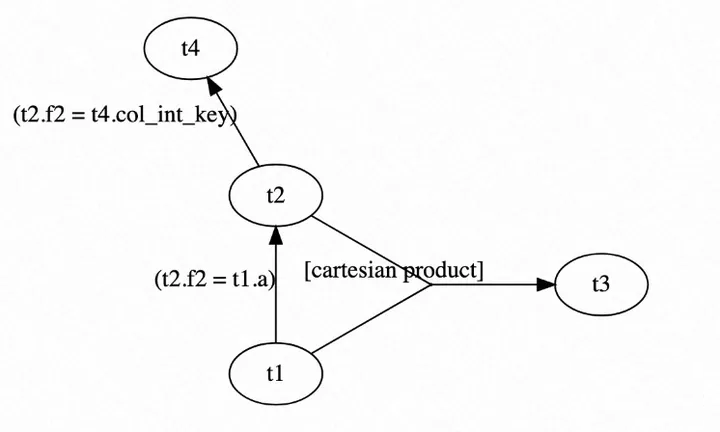0 1 0
Share on

# Related Products

• ##AnalyticDB for MySQL

AnalyticDB for MySQL is a real-time data warehousing service that can process petabytes of data with high concurrency and low latency.

• ##ApsaraDB RDS for MySQL

An on-demand database hosting service for MySQL with automated monitoring, backup and disaster recovery capabilities

• ##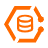ApsaraDB for MyBase

ApsaraDB Dedicated Cluster provided by Alibaba Cloud is a dedicated service for managing databases on the cloud.